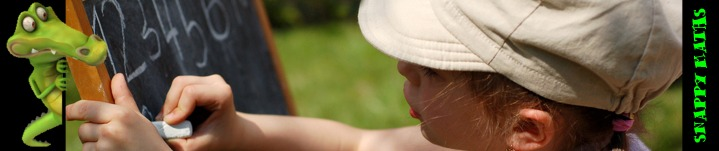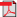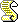﻿ Early Multiplication SkillsSnappy Maths (A Primary Topics Website) .
 Counting & Number Addition Subtraction Multiplication Division Mixed Bag Curriculum Other Stuff

## Early Multiplication Skills

 Please note: There seems to be some disagreement between educators when teaching early multiplication skills as to which part of the calculation represents the number in each group and which part represents the number of groups or whether this matters. We are hoping, eventually, to include resources for both so that you can choose those which fit with the system you work with.
 Our printable resourcesMultiplication Word ProblemsMultiplication Word Problems (reversed calculation)Multiplication Word Problems (Sheet 2)Multiplication Word Problems (Sheet 2 - reversed calculation)Repeated Addition (Mixed Pictures)Repeated Addition (Mixed Pictures - reversed calculation)Repeated Addition (Dice)Repeated Addition (Dice - reversed calculation)Repeated Addition (Cards)Repeated Addition (Cards - reversed calculation)Repeated Addition (Word Problems)Repeated Addition (Word Problems - reversed calculation)Multiplication Arrays (1)Multiplication Arrays (2)Multiplication Arrays (Word Problems 1)Multiplication Arrays (Word Problems 2)Multiplication Arrays (Word Problems 3)Multiplication Arrays (Word Problems 4)Our online activitiesRepeated Addition BingoMultiplication Array BingoMultiplication by Repeated Addition Interactive ActivityMultiplication Arrays Interactive ActivityHome | About Us | FAQs | Privacy | Contact Us
 All resources are for non-commercial, home or classroom use only.